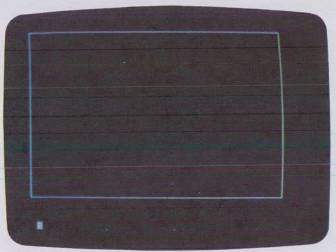LINE DRAWING

Another basic way to draw things on the computer involves using lines for these drawings instead of points. Lines are necessary when we wish to draw borders and boundaries, walls, bar graphs, simple geometric shapes, and so on. Certain computers allow you to draw a line anywhere on the screen with only one simple BASIC statement. We say these computers have vector graphics capability (the line is a vector). Some of the simple computers require that you make up a line as a series of dots, which is much harder but still possible.

Borders

Let's look at a simple example of how line-drawing statements work. To draw lines on the Apple we simply use a variation of the HPLOT statement called the HPLOT TO statement. Here is the syntax of the statement:

HPLOT x1, y1 TO x2, y2 (TO x3, y3 ..)

where x1, y1 represents the starting location of the line and x2, y2 represents the ending location of the line. The (TO x3, y3…) part means we can continue drawing lines to successive points by just adding more TO xN, yN statements to the main HPLOT. Note that this all assumes the color has been previously set with HCOLOR = . Recall that 0 < 279 and 0 < y < 159. See Example 8, p. 114.

Quite simple, isn't it? The coordinates in the HPLOT TO statement take us in a clockwise rotation around the screen starting at 0, 0 and ending at 0, 0 again.

Example 8:

Draw a continuous white border around the screen of the Apple using one long HPLOT TO statement.

Solution:

``` 10 HGR : HCOLOR = 3 20 HPLOT 0, 0 TO 279, 0 TO 279, 159 TO 0, 159 TO 0, 0 30 END ```

See Fig. 3-8.Fig. 3-8. Plot of continuous white border.

Diagonal Lines

Drawing diagonal lines allows us to make simple triangles and similar shapes. See Example 9, p. 115.

Here the first HPLOT statement selects the starting point for the line and then the successive HPLOT TO statements draw the triangle itself. We say the computer remembers the last coordinate location, so the next HPLOT TO statement causes the line to be drawn from this last location.